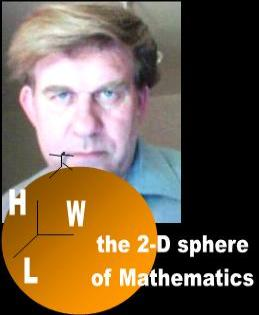A mathematician believes that planes are 3-D
 Adapted for the Internet from:Why God Doesn't Exist

Summary

Relativists invoke planes to introduce the uninitiated into the world of General Relativity. Specifically, they
use planes to contrast our 3-D universe against Abbott’s world of Flatland.  The flat world of planes helps
the neophyte visualize and contrast dimensional aspects of planes versus solids.

So what is a plane? Is a plane an idealized figure? Is it a figure? A real object? An abstract object? Do
planes exist? Is the plane the same thing as a surface? Does a plane have two dimensions? If not, how
many dimensions does it have? Do we have any use for planes in Physics?

It turns out that a mathematician talks about the 2-D world of Flatland, yet the scenarios he describes are
without exception 3-D. A mathematician also constructs his circles dynamically and claims that a triangle
has curved angles. He also tells you that he builds a cube by scanning a square. And so on. How can the
mathematicians assert that our Universe is four-dimensional when they don't even understand the two-
dimensional world of Flatland? The mathematicians never learned the first lesson of planes: there is no
such thing as a standalone plane. A 2-D geometric figure is necessarily part of a solid!

The misconceived definition of the word plane conduces the mathematicians to irrational conclusions.
Here I explore the infamous plane of Mathematics to get to the bottom of perhaps the most misunderstood
category of figures in Geometry. Specifically, I investigate the two planes that cause so much confusion to
the mathematicians: the triangle and the circle.2-D? Are you sure, Dr. Einstein? It seems to me that the little fellow is 3-D.________________________________________________________________________________________